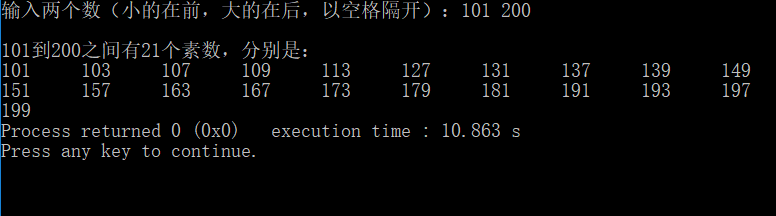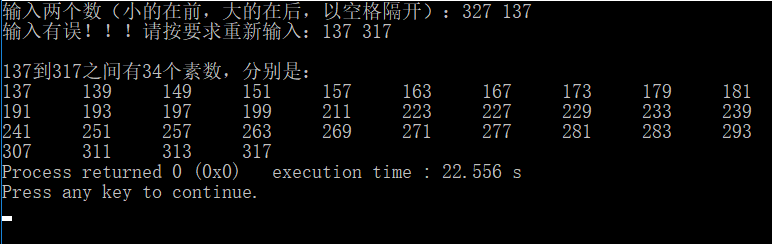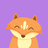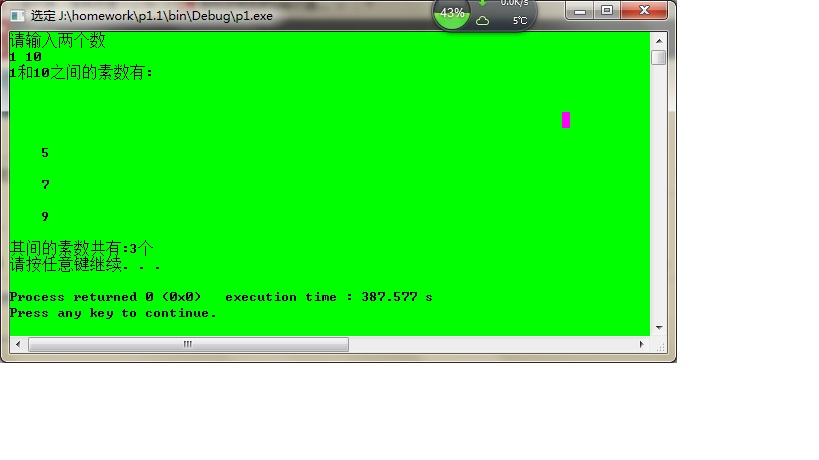• 从键盘任意输入两个整数，输出两数之间素数素数判断用函数实现。 扩展要求：1）程序可以循环执行，判断完一组可以再进行下一组；可以设定一个特殊键退出 2） 当输入的两个数不是大于2，则重新输入 3）输入的...c语言 c++
• 此程序为升级版，可以求用户输入的任意两个数之间素数，并将它们输出。 思路： 对两数之间的每一个进行从2到它自己减一的取余运算，从是否全不能整除来判断此是否是素数。 代码： #include &amp...

原题目：
求101到200之间的素数个数

• 此程序为升级版，可以求用户输入的任意两数之间的素数个数，并将它们输出。

思路：
对两数之间的每一个数进行从2到它自己减一的取余运算，从是否全不能整除来判断此数是否是素数。

代码：

#include <stdio.h>
#include <stdlib.h>

void find(int, int); //求素数个数的函数，参数为始末位置

void find(int num1, int num2)
{
int i = 0;
int j = 0;
int m = 0;   //计数器
int num = {0};     //存储素数
for(i = num1; i <= num2; i++)
{
j = 2;      //对因数j初始化
//用if语句将2的情况单独处理
if(i == 2)
num[m++] = 2;
while(i % j != 0 && i > j)  //判断是否符合条件
{
if(j == i - 1)	//如果所有的因子都符合条件
num[m++] = i;   //将符合条件的数据存储，计数器自增
j++;
}
}
printf("\n%d到%d之间有%d个素数，分别是：", num1, num2, m);
for(i = 0; num[i] != 0; i++)
{
if(i % 10 == 0)
printf("\n");
printf("%d\t", num[i]);
}

return 0;
}

int main()
{
int num1 = 0;
int num2 = 0;
printf("输入两个数（小的在前，大的在后，以空格隔开）：");
while(1)    //对输入的数据进行是否符合要求的判断
{
scanf("%d %d", &num1, &num2);
if(num1 < num2) //符合则跳出
break;
else            //不符合则重新输入
printf("输入有误！！！请按要求重新输入：");
}
find(num1, num2);

return 0;
}

运行结果：

• 输入101和200• 当输入的数据不符合条件时，提示重新输入**

• 题目练习，仅为一种思路，若有不足请指出。

**

展开全文求素数
• 小程序，可以方便找出两数之间质数，可以在c++6.0上打开
• 满意答案is_9527丶2013.11.13采纳率：45%等级：12已帮助：10113人供您参考：#include#include#define TRUE 1#define FALSE 0typedef int BOOL ;BOOL is_prime(int ptr);int main(){int start,end;...

满意答案is_9527丶

2013.11.13采纳率：45%    等级：12

已帮助：10113人

供您参考：

#include

#include

#define TRUE 1

#define FALSE 0

typedef int BOOL ;

BOOL is_prime(int ptr);

int main(){

int start,end;

int ptr;

int count=0;

printf("Input the range(start--end):");

scanf( "%d--%d",&start,&end);

for(ptr=start;ptr<=end;ptr++)

{

if(TRUE == is_prime(ptr))

{

count++;

printf("%d\t",ptr);

if(count % 5==0) printf("\n");

}

}

return 0;

}

BOOL is_prime(ptr)

{

int factor;

int sqr=sqrt(1.0*ptr);

for(factor=2;factor<=sqr ;factor++)

{

if(ptr % factor == 0 )

return FALSE;

}

return TRUE;

}

10分享举报

展开全文• 给你两个数，求两个数之间素数的个数。 输入： 输入有两个数，一个a，一个b，求a到b之间素数的个数 其中1 其中b-a 输出： 一个，表示a-b之间素数的个数。 样例输入： 1 10 样例输出： ...c++ c语言
• C程序显示两个数字之间的所有质数在此示例中，您将学习如何打印用户输入的两个数字之间的所有质数。要理解此示例，您应该了解以下C语言编程主题：显示两个间隔之间质数#includeintmain(){intlow,high,i,flag;...

C程序显示两个数字之间的所有质数

在此示例中，您将学习如何打印用户输入的两个数字之间的所有质数。

要理解此示例，您应该了解以下C语言编程主题：

显示两个间隔之间的质数#include

int main() {

int low, high, i, flag;

printf("输入两个数字(间隔): ");

scanf("%d %d", &low, &high);

printf("%d和%d之间的质数为: ", low, high);

//迭代直到low 不等于 high

while (low

flag = 0;

// 忽略小于2的数字

if (low <= 1) {

++low;

continue;

}

//如果low为非质数，则flag为1

for (i = 2; i <= low / 2; ++i) {

if (low % i == 0) {

flag = 1;

break;

}

}

if (flag == 0){

printf("%d ", low);

}

++low;

}

return 0;

}

输出结果输入两个数字(间隔): 20

50

20和50之间的质数为: 23 29 31 37 41 43 47

在此程序中，while循环被迭代(high-low-1)次。

在每次迭代中，检查low是否为质数，low的值增加1直到low等于high。

访问此页面以了解有关如何检查数字是否为质数的更多信息。

如果用户首先输入较大的数字，则上述程序将无法正常工作。您可以通过交换数字来解决此问题。

当较大的数字先输入时，显示质数#include

int main() {

int low, high, i, flag, temp;

printf("输入两个数字(间隔): ");

scanf("%d %d", &low, &high);

//如果low大于high则交换数字

if (low > high) {

temp = low;

low = high;

high = temp;

}

printf("%d和%d之间的质数为: ", low, high);

while (low

flag = 0;

//忽略小于2的数字

if (low <= 1) {

++low;

continue;

}

for (i = 2; i <= low / 2; ++i) {

if (low % i == 0) {

flag = 1;

break;

}

}

if (flag == 0)

printf("%d ", low);

++low;

}

return 0;

}

展开全文• 用Java编写一个程序，输出两个整数之间的所有素数
• 输出两个数之间的素数

千次阅读 2014-01-23 12:22:20
cout请输入两个数"; cin>>a>>b; cout和"之间素数有:"; for(c=a;c;c++) { prime=true; k=int(sqrt(c)); for(i=2;i;i++) { if(c%i==0) { prime=false; break; } if(prime) { cout(5); n=n+1; } ...
/*
* All rightsreserved.
* 文件名称： array.cpp
* 作    者：   王颖
* 完成日期：2013  年 1 月 23 日
* 版本号： v1.0
* 输入描述：无
*/
#include <iostream>
#include <iomanip>
#include<windows.h>
#include <cmath>
using namespace std;
int main()
{
int a,b,k,i,c=0,n=0;
bool prime;
cout<<"请输入两个数"<<endl;
cin>>a>>b;
cout<<a<<"和"<<b<<"之间的素数有:"<<endl;
for(c=a;c<=b;c++)
{
prime=true;
k=int(sqrt(c));
for(i=2;i<=k;i++)
{
if(c%i==0)
{
prime=false;
break;
}
if(prime)
{
cout<<setw(5)<<c;
n=n+1;
}
if(n%5==0)
cout<<endl;
}
cout<<endl;
}
cout<<"其间的素数共有:"<<n<<"个"<<endl;
system("pause");
return 0;
}展开全文• 则程序输出 2 3 5 7 11 13 17 19 #include using namespace std; int main() {  int a,b,i,j,s;  2a;  cin>>a>>b;    for(i=a;i  {  for(j=2;j  {  s=i%j;    if(s==0)C语言 程序设计 素数
• 目录能解决题目的代码并不是一次就...第一题：判断2-100之间有多少个素数，并输出所有素数。1.视频讲解：2.思路分析：q1:什么是素数？a1: 素数(质数)指的是在大于1的自然数中除了1和该本身外，无法被其它自然数...
• #include static int isPrime(int n); void main() { int low,high; int flag=1; while(flag==1) { printf("输出两个数（一空格分割）："); scanf("%d %d",&low,&high); /*
• Java程序显示两个间隔之间质数在该程序中，您将学习显示两个给定间隔(低和高)之间质数。您将学习在Java中使用while和for循环来做到这一点。示例：显示两个间隔之间质数publicclassPrime{publicstaticvoidmain...
• //判断一个数是不是素数 int checkPrimeNumber(int a) { int i,k;//循环、平方根、 int flag = 0;//标志 // 求平方根，注意sqrt()的参数为 double 类型， //这里要强制转换a的类型、还要注意这里的头文件哈 k =...c语言
• 输出从M到N之间的所有素数，每10数字占1行，其间以空格分隔，行末不可以有多余空格 M,N = map(int,input().split(' ')) X = [] for i in range(M,N+1): for j in range(2,i): if i % j == 0: break ...python
• 判断两个数之间的素数

千次阅读 2020-04-20 21:36:05
判断一个数是否为素数，求某个范围内的素数，以及算法的优化。python c语言 素数筛
• import java.util.*;public class Ex4_5 {public static void main(String[] args) {// TODO code application logic hereint a,b,i;...//定义一长为198的数组，因为这程序的意思是最大的200要分别除//以2-199...
• num1=int(input('请输入第一个数：')) num2=int(input('请输入第二个数：')) c=[] for a in range(num1,num2): i=2 while i&lt;a: if a%i==0: break i+=1 else: print(a,'是质数') ...Python
• printf("\n在%d到%d之间素数有：\n\n", a, b); for (i = a; i ; i++) { for (j = 2; j ; j++) { if (i % j == 0) break; } if (i == j) { n++; printf("%-5d", i); if (n % 5 == 0) ...c语言 判断素数
• #include <stdio.h> int main() { int m,n,j,cut,i,sum;...//输出两个整数，n是大于等于m的 cut = 0;// sum = 0;// for(i=m;i<=n;i++)//从m开始实验，一直检查到n，检查这个区间内是否有素数 ...
• #include int main() { int a,b; printf("please input two numbers \n"); scanf("%d %d",&a,&b); prime(a,b); return 0; } int prime(int x,int y) { int i,j,k,cout; cout=0; for(i=x;... k=C语言 求素数
• 【C语言】输入两输出两数之间的回文素数的个数 #include<stdio.h> //本例子仅仅适合于大于等于10以上的用例分析 int main() { int a,b,c; int i,sum = 0; int k,j = 0,y = 0,m; printf("输入两个数：...c语言
• **输出任意两个数之间的所有素数**import java.util.Scanner; public class Prime { public static void main(String[] args){ Scanner scan = new Scanner(System.in); System.out.println("下限：");class java小程序
• 输入任意两个数，满足10&lt;m&lt;n&lt;=3200，然后程序输出m~n之间所有素数测试程序如下：int m = 0, n = 0; int count = 0; //int temp = 0; bool NotSuShu ; cout&lt;&lt;"Please ...
• 结果图 1.引入包 import java.util.*; ...2.定义，获取输入 ... System.out.println("输入第一个数"); int x=input.nextInt(); System.out.println("输入第二个数"); int y=input.nextInt(); ...java 素数
• 例如输入1 100或者100 1，输出1到100之间素数，以及这些素数有多少，这些素数的和是多少。 #include <stdio.h> int isPrime(int num); int main(void) { int m, n; scanf("%d %d", &m, &...
• @python判断两个数之间质数数量 题目描述：输入两个数x,y 求区间[x,y]的质数数量 质数只有两个正因数（1和自己）的自然数即为质数。比1大但不是素数称为合数。1和0既非素数也非合数。特别注意：1不是质数。 ...python
• 代码粗糙，各路大神留下宝贵意见thx！ #include<stdio.h> #include<conio.h> int main( ) ... printf("请输入两个数：\n"); scanf("%d %d",&a,&b); if (a == 1 || a <= 0){a =
• package neida; import java.util.Scanner; public class output_prime { public static boolean isPrime(int i) { boolean tag = false;...i 内大专硕2018：输出两个整数之间的所有质数，并且每5个换行，假设2
• cout请输入两个数"; cin>>a>>b; cout和"之间素数有:"; for(c=a; c; c++) { prime=true; k=int(sqrt(c)); for(i=2; i; i++) if(c%i==0) { prime=false; break; } if(prime) { if(c>1) { cout(5);...
• 求两个数之间的素数

千次阅读 2018-05-30 20:38:13
求出min-max之间有多少个素数，并输出所有素数。 /** * 求出min-max之间有多少个素数，并输出所有素数。 * * @author cheng xin Li * */ public class Test { public static void main(String[] args) { ...Java Algorithm...